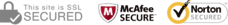The information provided on this section helps to analyse and visualise data based on Even and Odd numbers count.
• Even - Any number that can be divided exactly by 2 is an even number.
• Odd - Any number that cannot be divided exactly by 2 is an odd number.
From the below "Even and Odd set" the first number displayed is Even numbers count and the second number is the Odd numbers count. It shows information on the last 5 draws. Representation is also displayed as a Bar and Area chat diagram.
Even Odd Set Frequency
 [2, 4] 1 [3, 3] 2 [4, 2] 1 [5, 1] 1